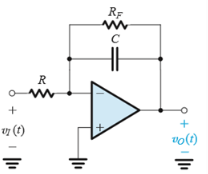Courses

# Test: Integrators & Differentiators

## 10 Questions MCQ Test Electronic Devices | Test: Integrators & Differentiators

Description
This mock test of Test: Integrators & Differentiators for Electrical Engineering (EE) helps you for every Electrical Engineering (EE) entrance exam. This contains 10 Multiple Choice Questions for Electrical Engineering (EE) Test: Integrators & Differentiators (mcq) to study with solutions a complete question bank. The solved questions answers in this Test: Integrators & Differentiators quiz give you a good mix of easy questions and tough questions. Electrical Engineering (EE) students definitely take this Test: Integrators & Differentiators exercise for a better result in the exam. You can find other Test: Integrators & Differentiators extra questions, long questions & short questions for Electrical Engineering (EE) on EduRev as well by searching above.
QUESTION: 1

### The other name for Miller Circuit is

Solution:

Miller Circuit is also called Inverting integrator.

QUESTION: 2

### The slope of the frequency response of an integrator is

Solution:

The slope is linear and negative.

QUESTION: 3

### The integrating transfer function has the value of

Solution:

Standard mathematical expression for the transfer function.

QUESTION: 4

The expression for the integration frequency is

Solution:

Standard mathematical expression for the integrator frequency.

QUESTION: 5

Determine the expression for the transfer function for the circuit shown below.​Solution:

It is a standard expression.

QUESTION: 6

The frequency transfer function of a differentiator is given by

Solution:

Standard mathematical expression for the transfer function of a differentiator.

QUESTION: 7

The slope of the frequency response of a differentiator is

Solution:

The slope is linear with a positive slope.

QUESTION: 8

The phase in the integrator and differentiator circuit respectively are

Solution:

These are the characteristics of the integrators and differentiators circuits respectively.

QUESTION: 9

Consider a symmetrical square wave of 20-V peak-to-peak, 0 average, and 2-ms period applied to a Miller integrator. Find the value of the time constant CR such that the triangular waveform at the output has a 20-V peak-to-peak amplitude.

Solution:

According to the question 1/CR = 2.

QUESTION: 10

The expression for the differentiator time constant is

Solution:

Standard mathematical expression for the time constant for the differentiators.